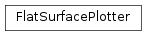# mvpa2.misc.plot.flat_surf.FlatSurfacePlotter¶class `mvpa2.misc.plot.flat_surf.``FlatSurfacePlotter`(surface, curvature=None, min_nsteps=500, range_='2_98%', threshold=None, color_map=None)

Plots data on a flat surface

Methods

 `set_underlay`(u) Sets the underlay
Parameters: surface: surf.Surface : a flat surface curvature: str or np.ndarray : (Filename of) data representing curvature at each node. min_steps: int : Minimal side of output plots in pixel range_: str or float or tuple : If a tuple (a,b), then this tuple is returned. If a float a, then (-a,a) is returned. “R(a)”, where R(a) denotes the string representation of float a, is equivalent to range_=a. “R(a)_R(b)” is equivalent to range_=(a,b). “R(a)_R(b)%” indicates that the a-th and b-th percentile of xs is taken to define the range. “R(a)%” is equivalent to “R(a)_R(100-a)%” threshold: str or float or tuple : Indicates which values will be shown. Syntax as in range_ color_map: str : colormap to use

Methods

 `set_underlay`(u) Sets the underlay
`set_underlay`(u)

Sets the underlay